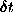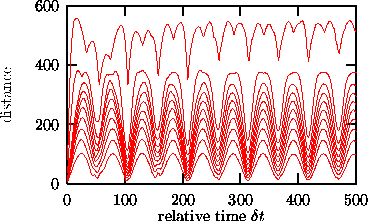Next: Nonlinear prediction Up: Visualizationnon-stationarity Previous: Recurrence plots

## Space-time separation plot

While the recurrence plot shows absolute times, the space-time separation plot introduced by Provenzale et al.  integrates along parallels to the diagonal and thus only shows relative times. One usually draws lines of constant probability per time unit of a point to be an-neighbor of the current point, when its time distance is. This helps identifying temporal correlations inside the time series and is relevant to estimate a reasonable delay time, and, more importantly, the Theiler-window w in dimension and Lyapunov-analysis (see Sec.). Said in different words, it shows how large the temporal distance between points should be so that we can assume that they form independent samples according to the invariant measure. The corresponding routine of the TISEAN package is stp, see Fig..Figure:   Space-time separation plot of the COlaser data. Shown are lines of constant probability density of a point to be-neighbor of the current point if its temporal distance is. Probability densitites are 1/10 to 1 with increments of 1/10 from bottom to top. Clear correlations are visible.Next: Nonlinear prediction Up: Visualizationnon-stationarity Previous: Recurrence plots

Thomas Schreiber
Wed Jan 6 15:38:27 CET 1999